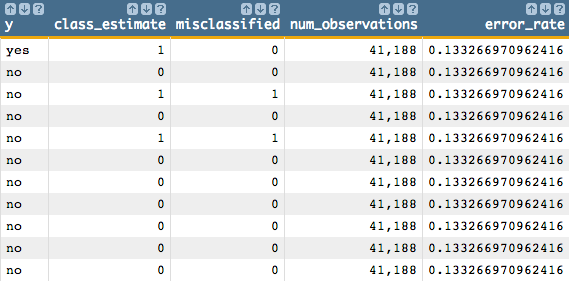# Clustering

In this example, clustering is used to separate a data set containing bank marketing information into two classes. The clustering results are then examined to see if they accurately reflect the underlying pattern in the data set, which in this example is whether or not a customer subscribed for a term deposit.

Clustering, using the 1010data function `g_cluster(G;S;XX;A;N;Z)`, will be performed on the Bank Marketing Data Set. This data set contains information related to a campaign by a Portuguese banking institution to get its customers to subscribe for a term deposit.

The clustering algorithm uses the following 10 variables in that data set:
• `age`
• `duration`
• `previous`
• `empvarrate`
• `housing`
• `default`
• `loan`
• `poutcome`
• `job`
• `marital`

Clustering is unsupervised learning, which means we assume there is no label for each observation. However, after running the clustering algorithm, we could compare the value of column `y` (whether someone subscribed for a term deposit) with our clustering results to see if our algorithm found the underlying pattern of data.

This analysis will follow the following steps:
• Prepare the data by creating dummy variables for each of the categorial columns (since we cannot use textual data to build our model).
• Run k-means clustering on the continuous variables in the original data set and the dummy variables that we created, partitioning the data into two classes.
• Classify the results of the clustering.
• Obtain the center of each class in various dimensions.
• Chart the two classes and plot their centers.
• Calculate the error rate by determining the percentage of observations that are misclassified.
1. Open the Bank Marketing data set (pub.demo.mleg.uci.bankmarketing).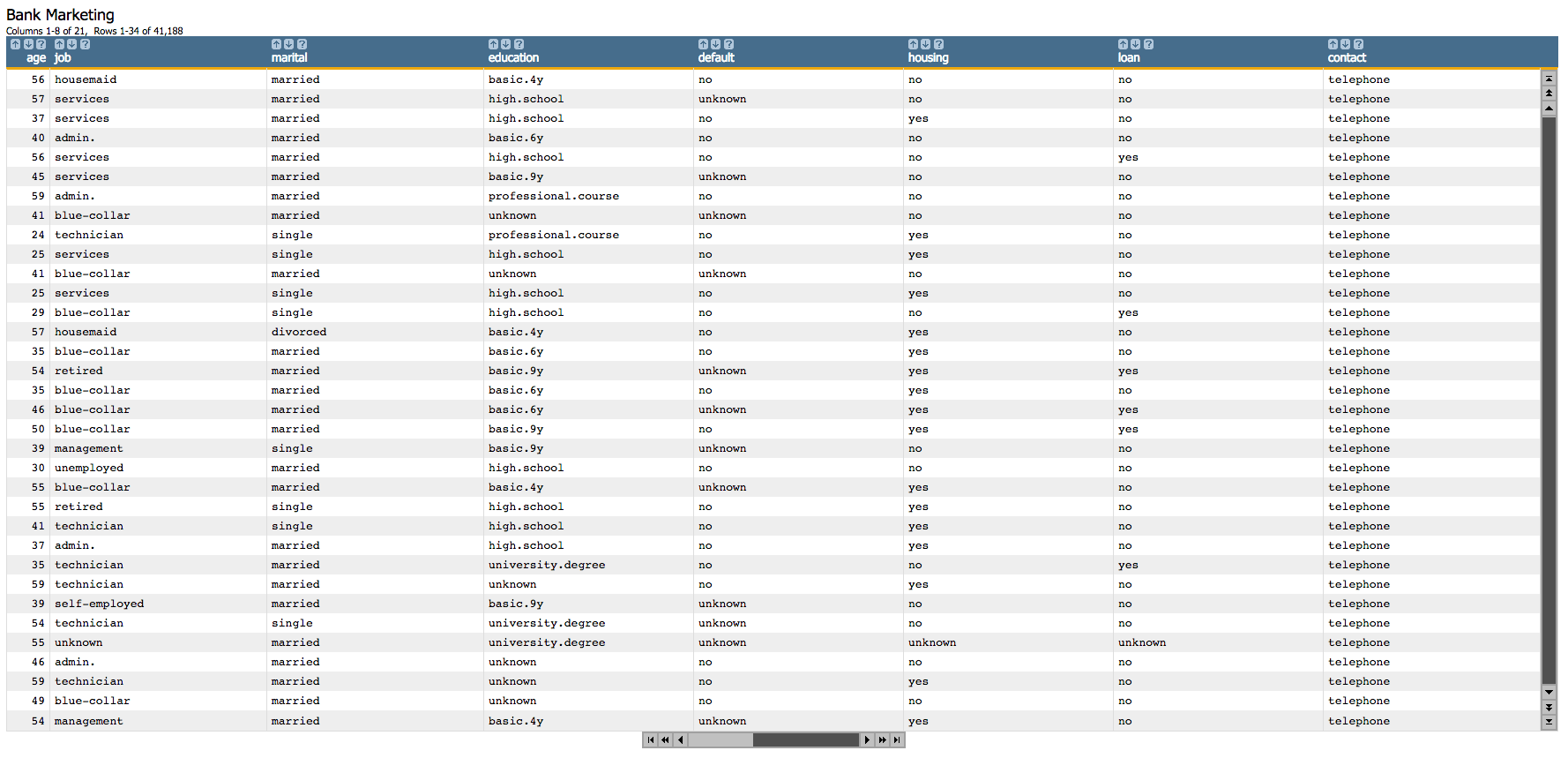2. Since we cannot use textual data in our analysis, we first create dummy variables for each of the categorial columns.
``````<willbe name="yy" value="y='yes'"/>
<willbe name="hsng" value="housing='yes'"/>
<willbe name="h_unk" value="housing='unknown'"/>
<willbe name="def" value="default='yes'"/>
<willbe name="d_unk" value="default='unknown'"/>
<willbe name="loans" value="loan='yes'"/>
<willbe name="l_unk" value="loan='unknown'"/>
<willbe name="nonxst" value="poutcome='nonexistent'"/>
<willbe name="succ" value="poutcome='success'"/>
<willbe name="blue" value="job='blue-collar'"/>
<willbe name="tech" value="job='technician'"/>
<willbe name="j_unk" value="job='unknown'"/>
<willbe name="svcs" value="job='services'"/>
<willbe name="mgmt" value="job='management'"/>
<willbe name="ret" value="job='retired'"/>
<willbe name="entr" value="job='entrepreneur'"/>
<willbe name="self" value="job='self-employed'"/>
<willbe name="maid" value="job='housemaid'"/>
<willbe name="unemp" value="job='unemployed'"/>
<willbe name="stud" value="job='student'"/>
<willbe name="marr" value="marital='married'"/>
<willbe name="sgl" value="marital='single'"/>
<willbe name="m_unk" value="marital='unknown'"/>``````

These `<willbe>` operations create a computed column for each of the categories, where a 1 in the column indicates that the category is true for that row. For instance, in the following screenshot, the rows where `hsng`=1 indicate that the client had a housing loan (i.e., `housing`='yes' in the original table), and the rows where `h_unk`=1 indicate that it is unknown if the client had a housing loan (i.e., `housing`='unknown').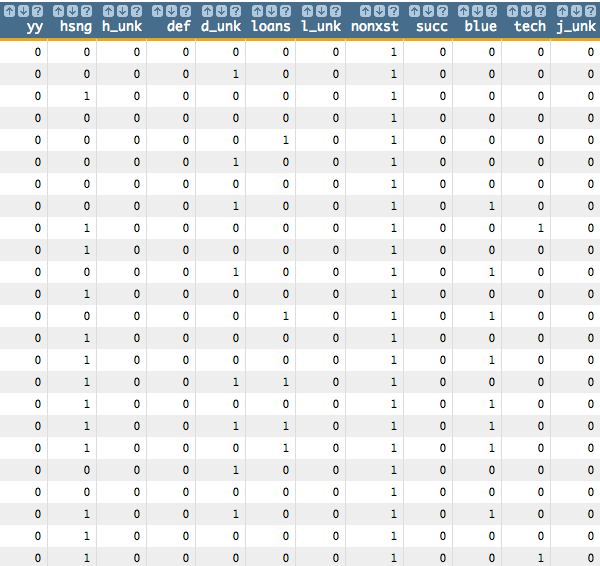See Dummy Variables for a list of the dummy variables used here and their meanings.

3. Using `g_cluster(G;S;XX;A;N;Z)`, we run k-means clustering on the continuous variables in the original data set and the dummy variables that we created. Using the `N` argument, we specify that we want to partition the data into two classes.
``````<note>CLUSTER WITH 26 VARIABLES</note>
<willbe name="model_cluster" value="g_cluster(;;age duration previous
empvarrate hsng h_unk def d_unk loans l_unk nonxst succ blue tech
j_unk svcs mgmt ret entr self maid unemp stud marr sgl m_unk;'kmeans';2;)"/>``````

This creates a column named `model_cluster` that contains the results of the clustering: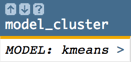Clicking on the `>` opens a window containing a summary of the model output: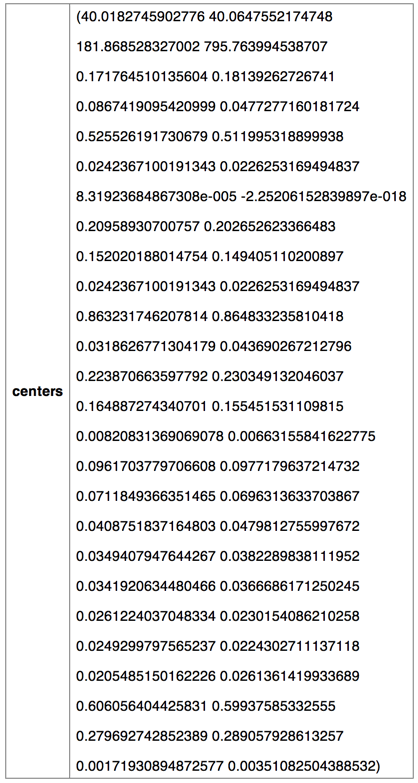4. We can then classify the results of the clustering by using the `classify(XX;M;Z)` function.
``````<willbe name="class_estimate" value="classify(age duration previous
empvarrate hsng h_unk def d_unk loans l_unk nonxst succ blue tech j_unk
svcs mgmt ret entr self maid unemp stud marr sgl m_unk;model_cluster;)"/>``````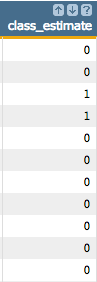The two classes that result from our clustering algorithm have the values 0 and 1 in the `class_estimate` column.

5. Let's obtain the center of each class for the various dimensions (e.g., `age`, `duration`, `previous`) using the `param(M;P;I)` function.

For instance, `param(model_cluster;'centers';2 1)` gives us the second dimension of the first class. (In our example, the second dimension is `duration`.)

So the following will give us the first three dimensions of both classes:

``````<willbe name="center_class_1_1" value="param(model_cluster;'centers';1 1)"/>
<willbe name="center_class_2_1" value="param(model_cluster;'centers';2 1)"/>
<willbe name="center_class_3_1" value="param(model_cluster;'centers';3 1)"/>
<willbe name="center_class_1_2" value="param(model_cluster;'centers';1 2)"/>
<willbe name="center_class_2_2" value="param(model_cluster;'centers';2 2)"/>
<willbe name="center_class_3_2" value="param(model_cluster;'centers';3 2)"/>``````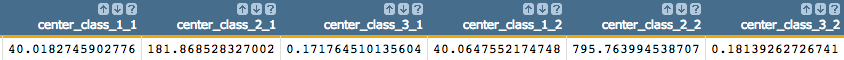6. Finally, we can visualize the results of the clustering algorithm using the 1010data Chart Builder.
1. Create two columns for each class corresponding to the `age` and `duration` columns.
Note: Rows that contain N/A values will not be charted in 1010data.
``````<willbe name="cluster_1_age" value="if(class_estimate=0;age;NA)"/>
<willbe name="cluster_1_duration" value="if(class_estimate=0;duration;NA)"/>
<willbe name="cluster_2_age" value="if(class_estimate=1;age;NA)"/>
<willbe name="cluster_2_duration" value="if(class_estimate=1;duration;NA)"/>``````
2. Click Chart > Scatter.
3. To visualize the first class, drag the `cluster_1_age` column to the DATA (X-AXIS) area and `cluster_1_duration` to the DATA (Y-AXIS) area.
4. To visualize the second class, drag `cluster_2_age` to DATA (X-AXIS) and `cluster_2_duration` to DATA (Y-AXIS).
5. To plot the center of the first class, drag `center_class_1_1` to DATA (X-AXIS) and `center_class_2_1` to DATA (Y-AXIS).
6. To plot the center of the second class, drag `center_class_1_2` to DATA (X-AXIS) and `center_class_2_2` to DATA (Y-AXIS).
7. To set the colors for the classes and their centers, under the DATA SERIES section of the Customization Settings panel, enter the following in the Colors field: #c0504d;#000000;#c0504d;#000000
8. To set the scatter point sizes for the classes and their centers, under the DATA SERIES section of the Customization Settings panel, enter the following in the Scatter point sizes field: small,small,large,large
9. Click Update.

The results should look similar to the following chart: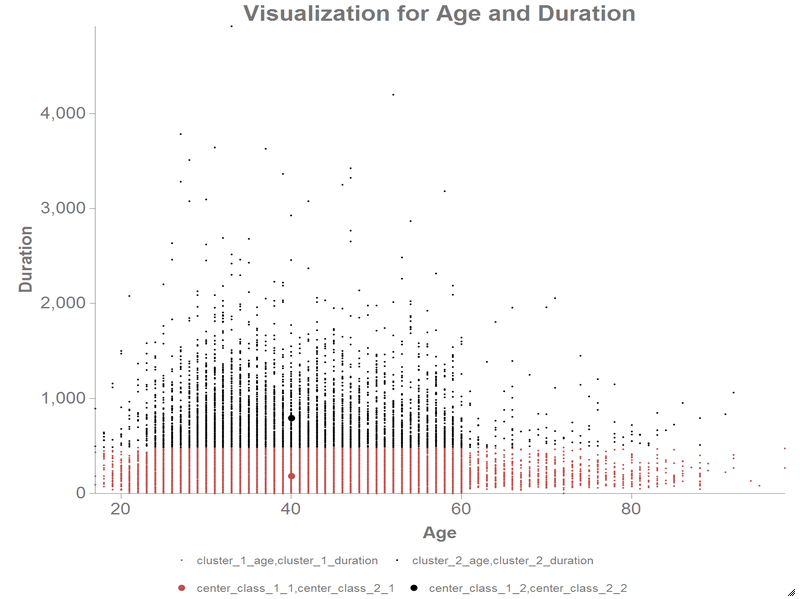In the above chart, the red scatter points represent one class, and the black scatter points represent the other. The larger red and black scatter points represent the centers of those classes.

7. We can calculate the error rate by determining the percentage of observations that are misclassified; that is, we can compare the clustering results to see if they accurately reflect the underlying pattern in the data set, which in our example is whether a customer subscribed for a term deposit or not.

It looks like, for the most part, the cluster with the value 0 for `class_estimate` looks like it matches up with those values in `y` whose values are no, and the cluster with the value 1 for `class_estimate` looks like it matches up with those values in `y` whose values are yes.

Let's find the number of observations in which neither of these cases is true to flag those observations as misclassified. Then we'll divide that number by the total number of observations to find the error rate.

``````<willbe name="misclassified" value="(y='no'&class_estimate=1)|(y='yes'&class_estimate=0)"/>
<willbe name="num_observations" value="n_"/>
<willbe name="error_rate" value="g_cnt(;misclassified)/num_observations"/>``````